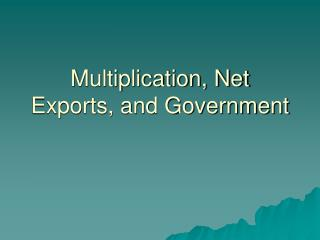DownloadDownload PresentationMultiplication, Net Exports, and Government

# Multiplication, Net Exports, and Government

Télécharger la présentation## Multiplication, Net Exports, and Government

- - - - - - - - - - - - - - - - - - - - - - - - - - - E N D - - - - - - - - - - - - - - - - - - - - - - - - - - -
##### Presentation Transcript

1. Multiplication, Net Exports, and Government

2. I. Multiplier • Multiplier effect: a change in a component of agg expenditures leads to a larger change in equilibrium GDP • Multiplier = change rGDP/initial change in spending • 1) initial change usually investment (more volatile), but can be C,G, I, X • 2) initial change: up or downshift in agg expend schedule due to up or downshift in component • 3) multiplier can be negative

3. Source of Multiplication • 1) repetitive, continuous flow of money • 2) change income fractional, same direction change in S and C •  diminishing spending chain • ∆I ∆wage, rent, interest, profit (spending and receiving income sides same coin) • Multiplication ends when ∆I matched by exactly offsetting S: corrects disequilibrium

4. MPC and MPS • Amount of multiplication necessary to recreate equilibrium determined by MPC and MPS • Multiplier = 1/MPS • MPS + MPC = 1 • Multipliers = 1/(1-MPC)

5. Significance and Generalization • Multiplier creates larger fluctuations in economy from biz decisions: greater booms, greater busts • Simple multiplier: leakage only into savings (MPS of .05 = 20x) • Complex: leakage into taxes, imports (“fraction of the change in income which leaks, or is diverted, from the income-expenditure stream”); estimated at 2x

6. II. Trade and Equilibrium Output • C + I = private closed economy agg expend • C + I + (X-M)= private open economy • Net exports increase agg expenditure beyond what closed is capable of, so GDP up; net imports reduce agg ex below closed level, so GDP down • HOWEVER…

7. AS determined by input prices • Int’l trade generally increases efficiency •  lower costs of production (of all resources, including investment capital (“money” is the single largest traded “good”)) •  greater output • Therefore, GDP higher in open economy • Also, int’l trade increases international incomes, therefore higher levels of exports (partly offsets lower GDP from imports) • Empirical evidence: “beggar-thy-neighbor” tariff wars of 1920s-30s

8. Exchange rates • Depreciation: dollar losing value (inflation) • Effect on net exports? • Appreciation: dollar gaining value (dis- or deflation) • Effect on net exports? • Effect on GDP? • Where are we on the curve?

9. III. Government • C + I + G + (X-M) • 1) Continue simplified investment and net export schedules • 2) G no effect on private spending • 3) Assume tax revenue entirely personal taxes; DI<PI; but GDP=NI=PI • 4) Fixed level of taxes regardless of GDP • 5) Price level constant

10. Spend and Tax • G spending increases agg expenditures and equilibrium GDP by a multiplier • G is an injection offsetting S and M • No, that’s not what I mean • G taxing reduces ae and eGDP by a multiplier, but indirectly by reducing DI C; tax multiplier = tax x MPC • G taxes are a leakage • Tax cuts increase ae and eGDP, but less than an increase in spending

11. Balanced Budget Multiplier • \$20 B in spending + \$20 B in higher taxes • Increase spending: AE increases by full \$20 B • Increase taxes: AE decreases by 20 x MPC= 20(.95)= 19 • Net ∆ AE = 1 • 1 x (1/1-.95) = \$20 B ∆ GDP • Balanced budget multiplier is always 1